# [HowTo] LINQ - First Start # Range Operator

This HowTo introduces how to use the Range operator.

# Introduction

The range operator can be used to generate a consecutive sequence of integers.

## Example

I – Input P – Processing O – Output
Start: 0, Length: 5 Generate Sequence starting from 0 with a length of 5 {0,1,2,3,4}
Start: 5, Length: 5 Generate Sequence as an Integer Array
starting from 5 with a length of 5
{5,6,7,8,9}
Start: -3, Length: 5 Generate Sequence as an integer List
starting from -3 with a length of 5
{-3,-2,-1,0,1}

# Implementation

## Method Syntax

``````mySequence  = Enumerable.Range(0,5)             // returned Datatype: IEnumerable(Of Int32)
arrSequence  = Enumerable.Range(5,5).ToArray()  // returned Datatype: Int32()
listSequence= Enumerable.Range(-3,5).ToList()   // returned DataType: List(of Int32)
``````
Visuals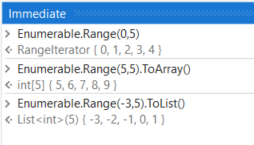## Query Syntax

The Range operator is not available as a clause for the query syntax

# Examples of use

In addition to the application scenarios that generate a simple sequence of ascending integers, further cases can be implemented in combination with other LINQ operators or advanced statements.

## Descending sequence

``````Enumerable.Range(-10,11).Reverse()
``````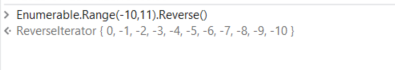## Sequence with even numbers in a range

``````Enumerable.Range(1,10).Where(Function (x) x Mod 2 = 0)
``````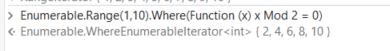## Sequence with capital letters

``````Enumerable.Range(65,26).Select(Function (x) Chr(x).ToString())
``````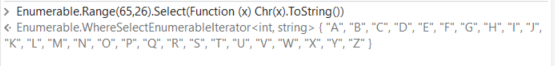## All dates from this month

``````Enumerable.Range(0, DateTime.DaysInMonth(now.year, now.Month)).Select(Function (x) new DateTime(now.Year, now.Month,1).addDays(x).Date)
``````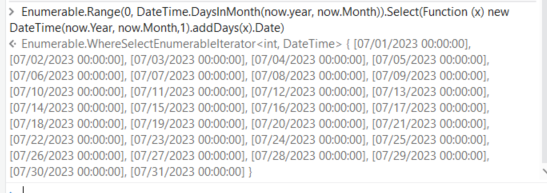# Questions

For questions on your use case open a new topic and get individual support

2 Likes